# Volumes of Cuboids and Prisms

In this worksheet, students calculate the volumes of cuboids and prisms.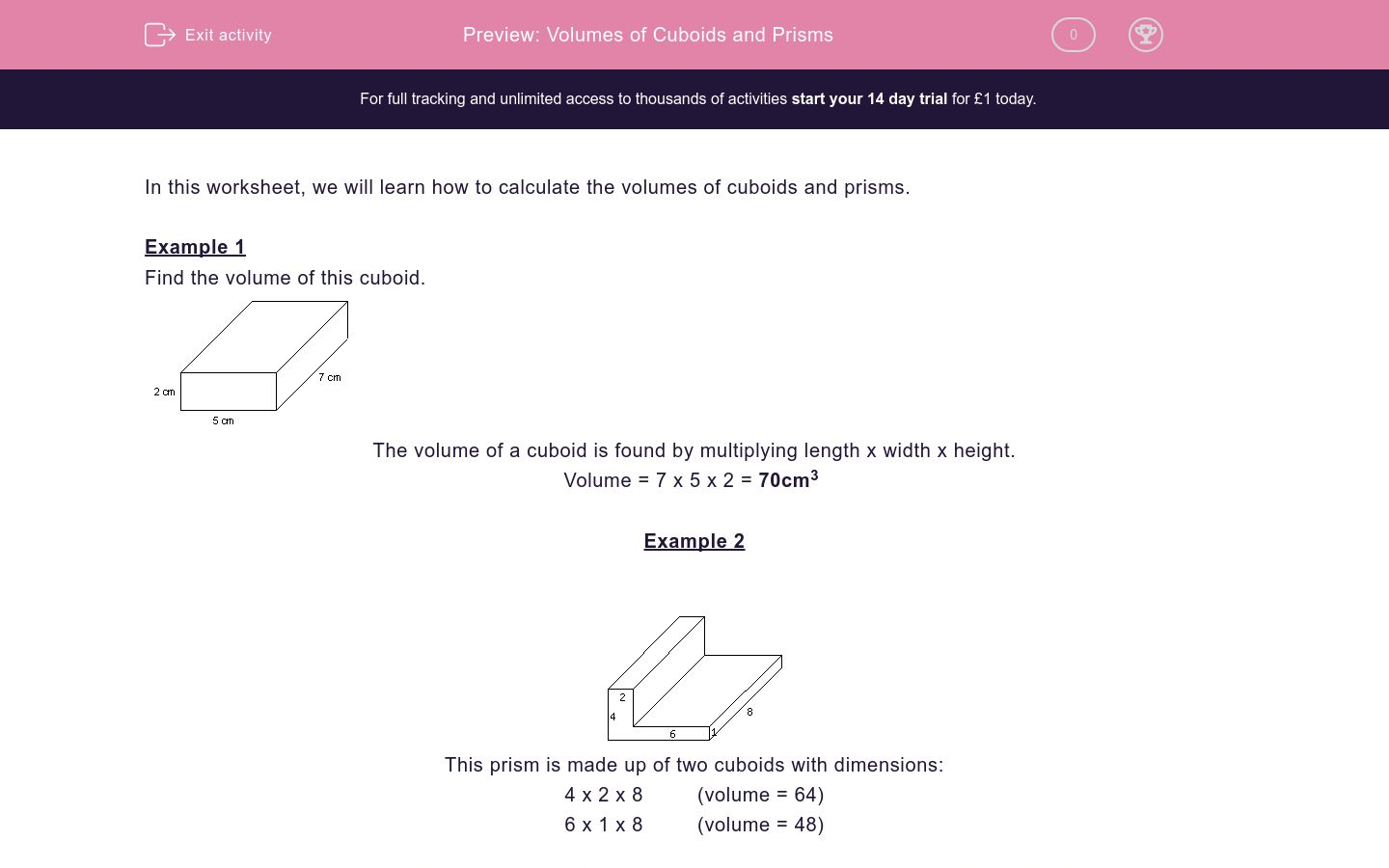Key stage:  KS 2

Curriculum topic:   Maths and Numerical Reasoning

Curriculum subtopic:   Volume and Capacity

Difficulty level:### QUESTION 1 of 10

In this worksheet, we will learn how to calculate the volumes of cuboids and prisms.

Example 1

Find the volume of this cuboid.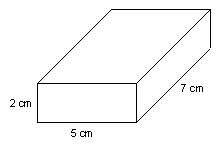The volume of a cuboid is found by multiplying length x width x height.

Volume = 7 x 5 x 2 = 70cm3

Example 2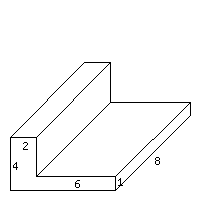This prism is made up of two cuboids with dimensions:

4 x 2 x 8         (volume = 64)

6 x 1 x 8         (volume = 48)

The volume of the prism can be found by adding together the volumes of the cuboids.

Volume of prism = 64 + 48 = 112 cu. units.

Find the volume of the following prism by splitting it into two cuboids and adding the volumes together.

(Just write the number)Find the volume of the following prism by splitting it into two cuboids and adding the volumes together.

(Just write the number)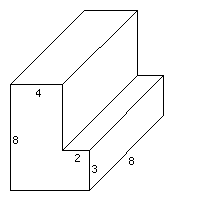Find the volume of the following prism by splitting it into two cuboids and adding the volumes together.

(Just write the number)Find the volume of the following prism by splitting it into two cuboids and adding the volumes together.

(Just write the number)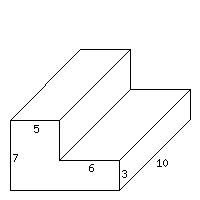Find the volume of the following prism by splitting it into two cuboids and adding the volumes together.

(Just write the number)Find the volume of the following prism by splitting it into two cuboids and adding the volumes together.

(Just write the number)Find the volume of the following prism by splitting it into two cuboids and adding the volumes together.

(Just write the number)Find the volume of the following prism by splitting it into two cuboids and adding the volumes together.

(Just write the number)Find the volume of the following prism by splitting it into two cuboids and adding the volumes together.

(Just write the number)Find the volume of the following prism by splitting it into two cuboids and adding the volumes together.

(Just write the number)• Question 1

Find the volume of the following prism by splitting it into two cuboids and adding the volumes together.

(Just write the number)120
• Question 2

Find the volume of the following prism by splitting it into two cuboids and adding the volumes together.

(Just write the number)304
• Question 3

Find the volume of the following prism by splitting it into two cuboids and adding the volumes together.

(Just write the number)259
• Question 4

Find the volume of the following prism by splitting it into two cuboids and adding the volumes together.

(Just write the number)530
• Question 5

Find the volume of the following prism by splitting it into two cuboids and adding the volumes together.

(Just write the number)108
• Question 6

Find the volume of the following prism by splitting it into two cuboids and adding the volumes together.

(Just write the number)210
• Question 7

Find the volume of the following prism by splitting it into two cuboids and adding the volumes together.

(Just write the number)243
• Question 8

Find the volume of the following prism by splitting it into two cuboids and adding the volumes together.

(Just write the number)378
• Question 9

Find the volume of the following prism by splitting it into two cuboids and adding the volumes together.

(Just write the number)112
• Question 10

Find the volume of the following prism by splitting it into two cuboids and adding the volumes together.

(Just write the number)182
---- OR ----

Sign up for a £1 trial so you can track and measure your child's progress on this activity.

### What is EdPlace?

We're your National Curriculum aligned online education content provider helping each child succeed in English, maths and science from year 1 to GCSE. With an EdPlace account you’ll be able to track and measure progress, helping each child achieve their best. We build confidence and attainment by personalising each child’s learning at a level that suits them.

Get started